# 树莓派 4 上的实时推理 （30 FPS！）

PyTorch对Raspberry Pi 4有开箱即用的支持。本教程将指导您如何设置Raspberry Pi 4以运行PyTorch并在CPU上运行MobileNet v2 的实时分类模型 （30 fps+）。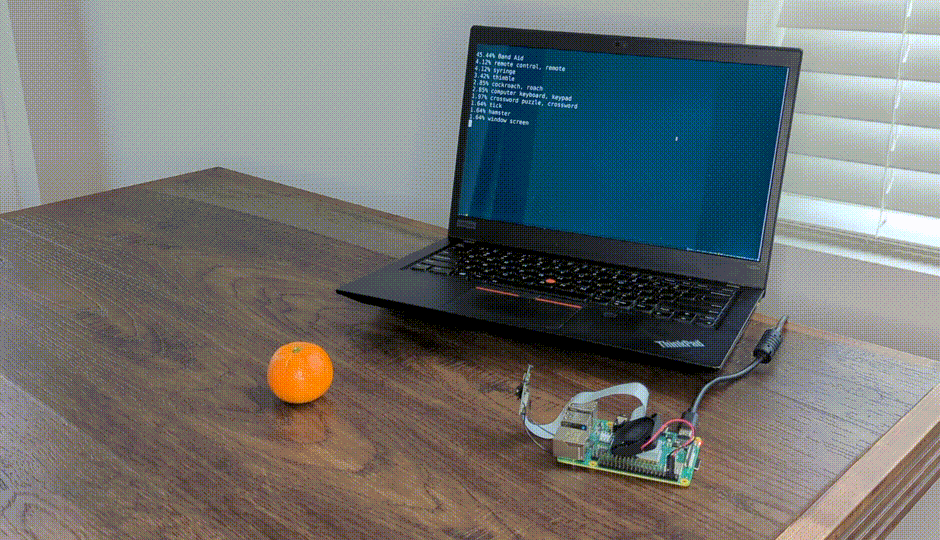# 树莓派 4 设置

PyTorch只为Arm 64位（aarch64）提供pip包，所以你需要在Raspberry Pi上安装64位版本的操作系统。

32位树莓派操作系统将无法工作。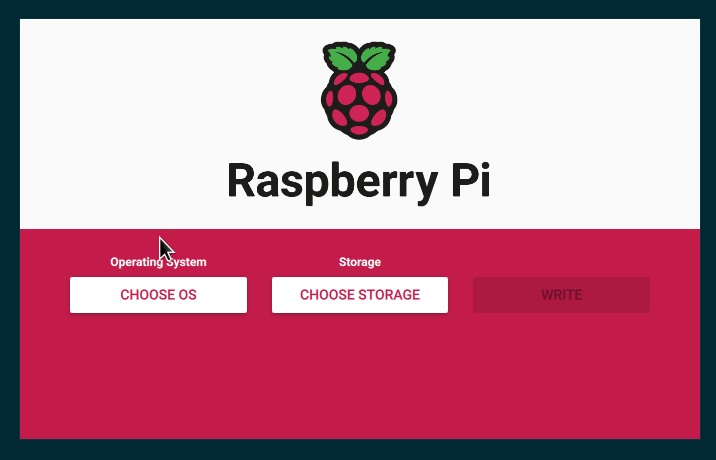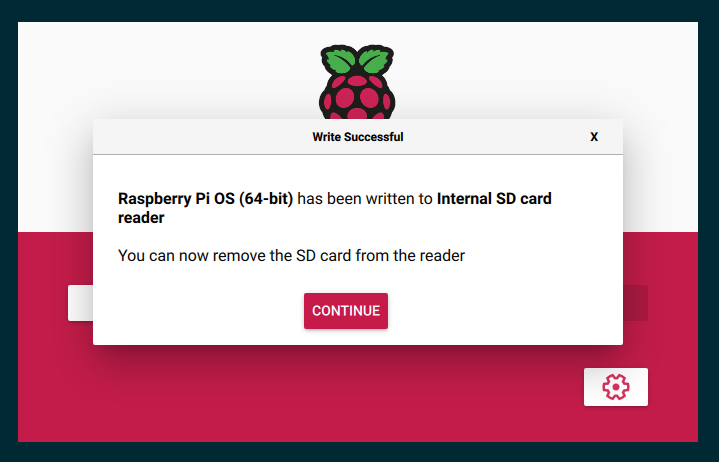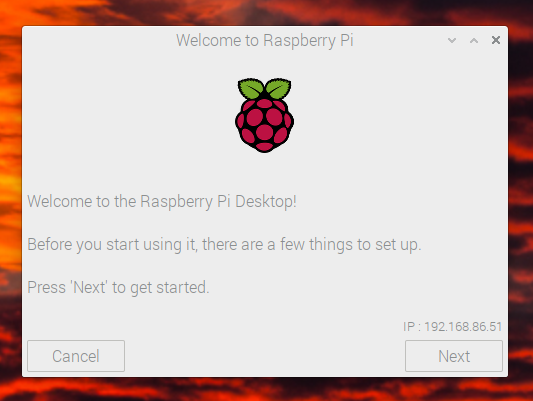# This enables the extended features such as the camera.
start_x=1

# This needs to be at least 128M for the camera processing, if it's bigger you can just leave it as is.
gpu_mem=128

# You need to commment/remove the existing camera_auto_detect line since this causes issues with OpenCV/V4L2 capture.
#camera_auto_detect=1


# 安装 PyTorch 和 OpenCV

$pip install torch torchvision torchaudio$ pip install opencv-python
$pip install numpy --upgrade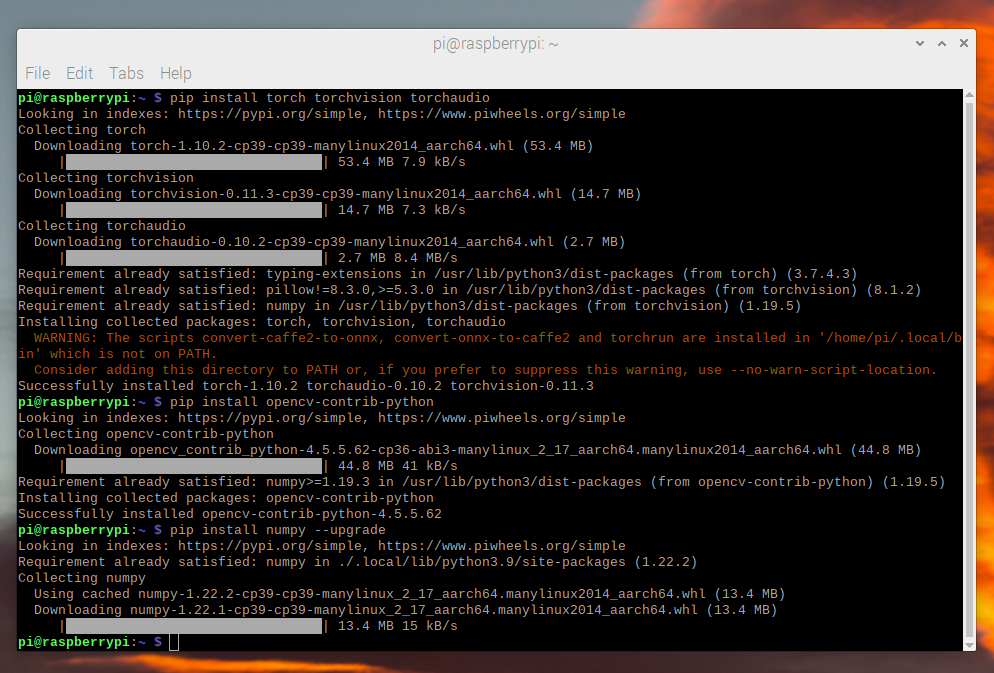现在我们可以检查所有内容是否正确安装： $ python -c "import torch; print(torch.__version__)"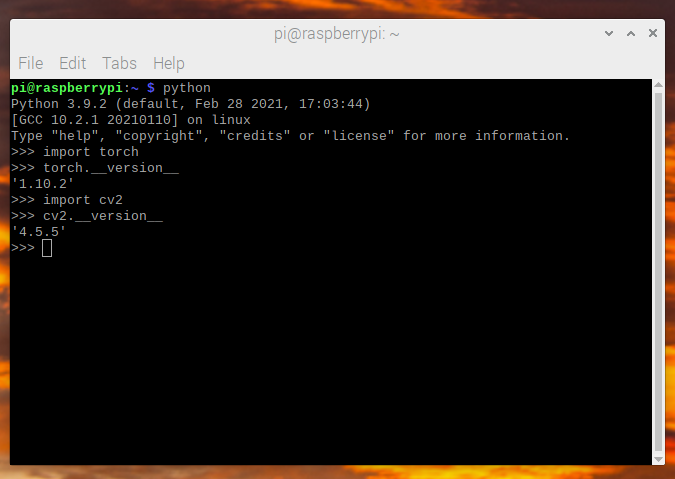# 视频捕获

import cv2
from PIL import Image

cap = cv2.VideoCapture(0)
cap.set(cv2.CAP_PROP_FRAME_WIDTH, 224)
cap.set(cv2.CAP_PROP_FRAME_HEIGHT, 224)
cap.set(cv2.CAP_PROP_FPS, 36)


OpenCV 在 BGR 中返回一个numpy数组，因此我们需要读取并执行一些随机播放以使其转换为预期的 RGB 格式。

ret, image = cap.read()
# convert opencv output from BGR to RGB
image = image[:, :, [2, 1, 0]]


# 图像预处理

from torchvision import transforms

preprocess = transforms.Compose([
# convert the frame to a CHW torch tensor for training
transforms.ToTensor(),
# normalize the colors to the range that mobilenet_v2/3 expect
transforms.Normalize(mean=[0.485, 0.456, 0.406], std=[0.229, 0.224, 0.225]),
])
input_tensor = preprocess(image)
# The model can handle multiple images simultaneously so we need to add an
# empty dimension for the batch.
# [3, 224, 224] -> [1, 3, 224, 224]
input_batch = input_tensor.unsqueeze(0)


# Model选择

Model FPS Total Time (ms/frame) Model Time (ms/frame) qnnpack Pretrained
mobilenet_v2 33.7 29.7 26.4 True
mobilenet_v3_large 29.3 34.1 30.7 True
resnet18 9.2 109.0 100.3 False
resnet50 4.3 100.3 225.2 False
resnext101_32x8d 1.1 892.5 885.3 False
inception_v3 4.9 204.1 195.5 False
shufflenet_v2_x0_5 46.7 21.4 18.2 False
shufflenet_v2_x1_0 24.4 41.0 37.7 False
shufflenet_v2_x1_5 16.8 59.6 56.3 False
shufflenet_v2_x2_0 11.6 86.3 82.7 False

# MobileNetV2：量化和 JIT

aarch64版本的pytorch需要使用qnnpack引擎。

import torch
torch.backends.quantized.engine = 'qnnpack'


from torchvision import models
net = models.quantization.mobilenet_v2(pretrained=True, quantize=True)


net = torch.jit.script(net)


# 把它放在一起

import time

import torch
import numpy as np
from torchvision import models, transforms

import cv2
from PIL import Image

torch.backends.quantized.engine = 'qnnpack'

cap = cv2.VideoCapture(0, cv2.CAP_V4L2)
cap.set(cv2.CAP_PROP_FRAME_WIDTH, 224)
cap.set(cv2.CAP_PROP_FRAME_HEIGHT, 224)
cap.set(cv2.CAP_PROP_FPS, 36)

preprocess = transforms.Compose([
transforms.ToTensor(),
transforms.Normalize(mean=[0.485, 0.456, 0.406], std=[0.229, 0.224, 0.225]),
])

net = models.quantization.mobilenet_v2(pretrained=True, quantize=True)
# jit model to take it from ~20fps to ~30fps
net = torch.jit.script(net)

started = time.time()
last_logged = time.time()
frame_count = 0

while True:
if not ret:

# convert opencv output from BGR to RGB
image = image[:, :, [2, 1, 0]]
permuted = image

# preprocess
input_tensor = preprocess(image)

# create a mini-batch as expected by the model
input_batch = input_tensor.unsqueeze(0)

# run model
output = net(input_batch)
# do something with output ...

# log model performance
frame_count += 1
now = time.time()
if now - last_logged > 1:
print(f"{frame_count / (now-last_logged)} fps")
last_logged = now
frame_count = 0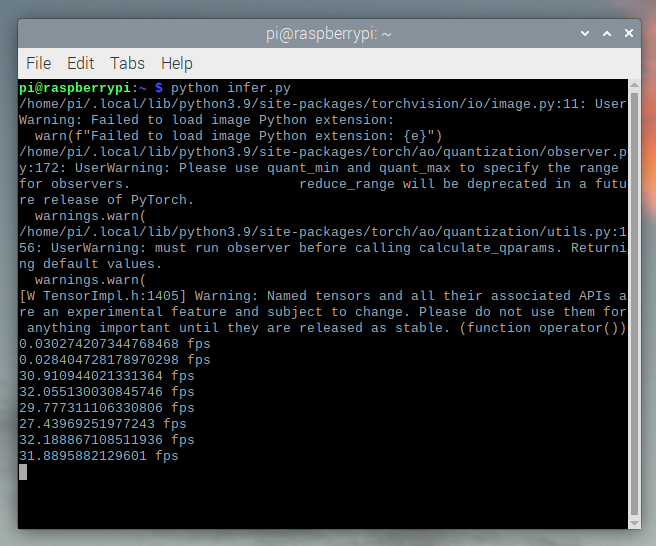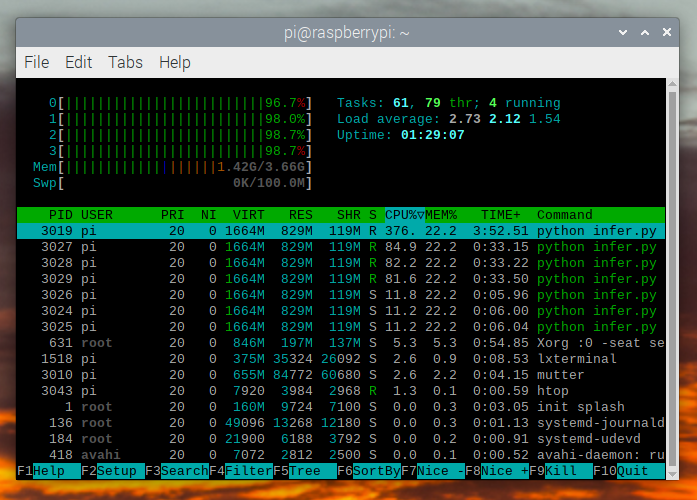top = list(enumerate(output.softmax(dim=0)))
top.sort(key=lambda x: x, reverse=True)
for idx, val in top[:10]:
print(f"{val.item()*100:.2f}% {classes[idx]}")


mobilenet_v3_large实时运行：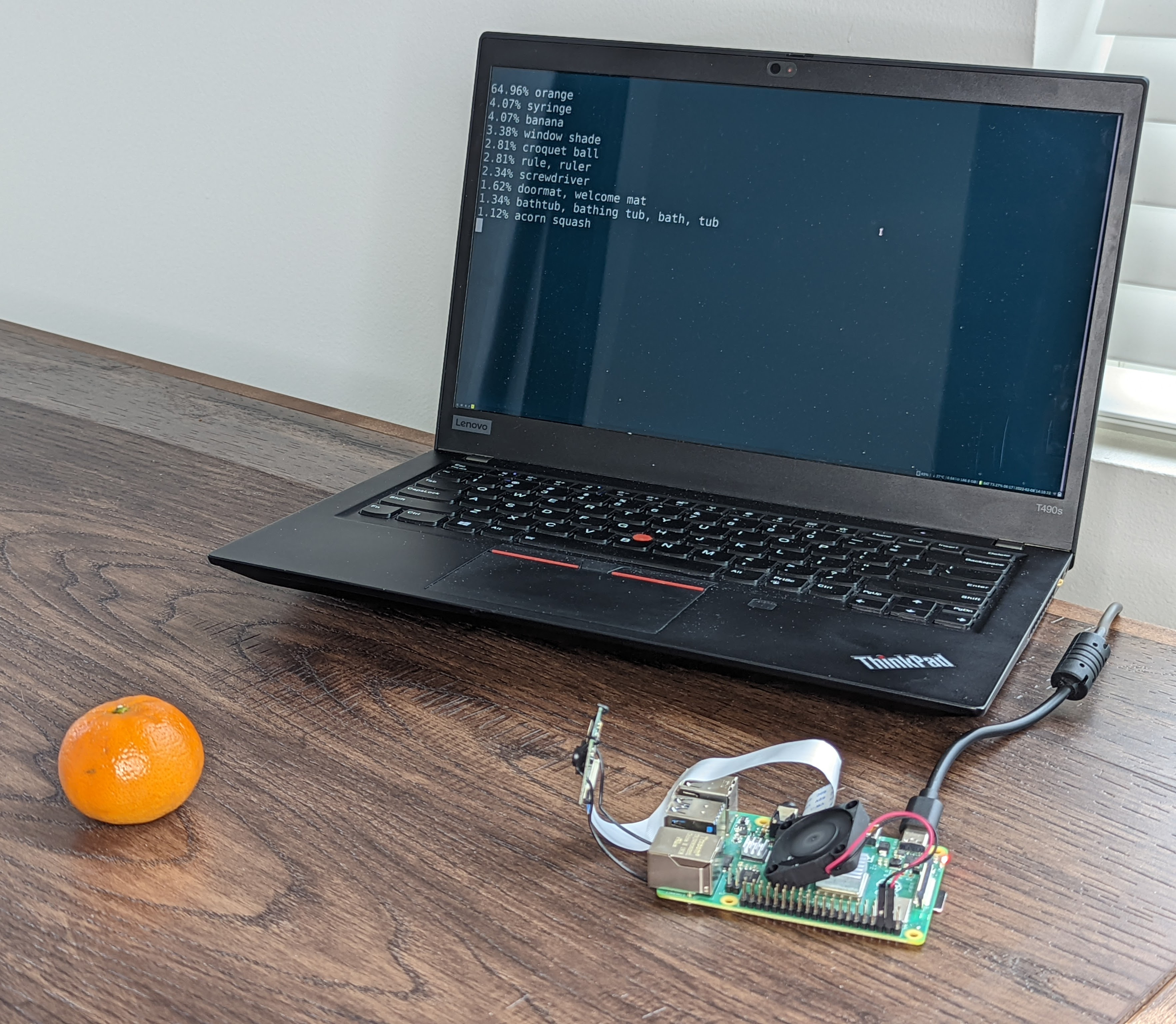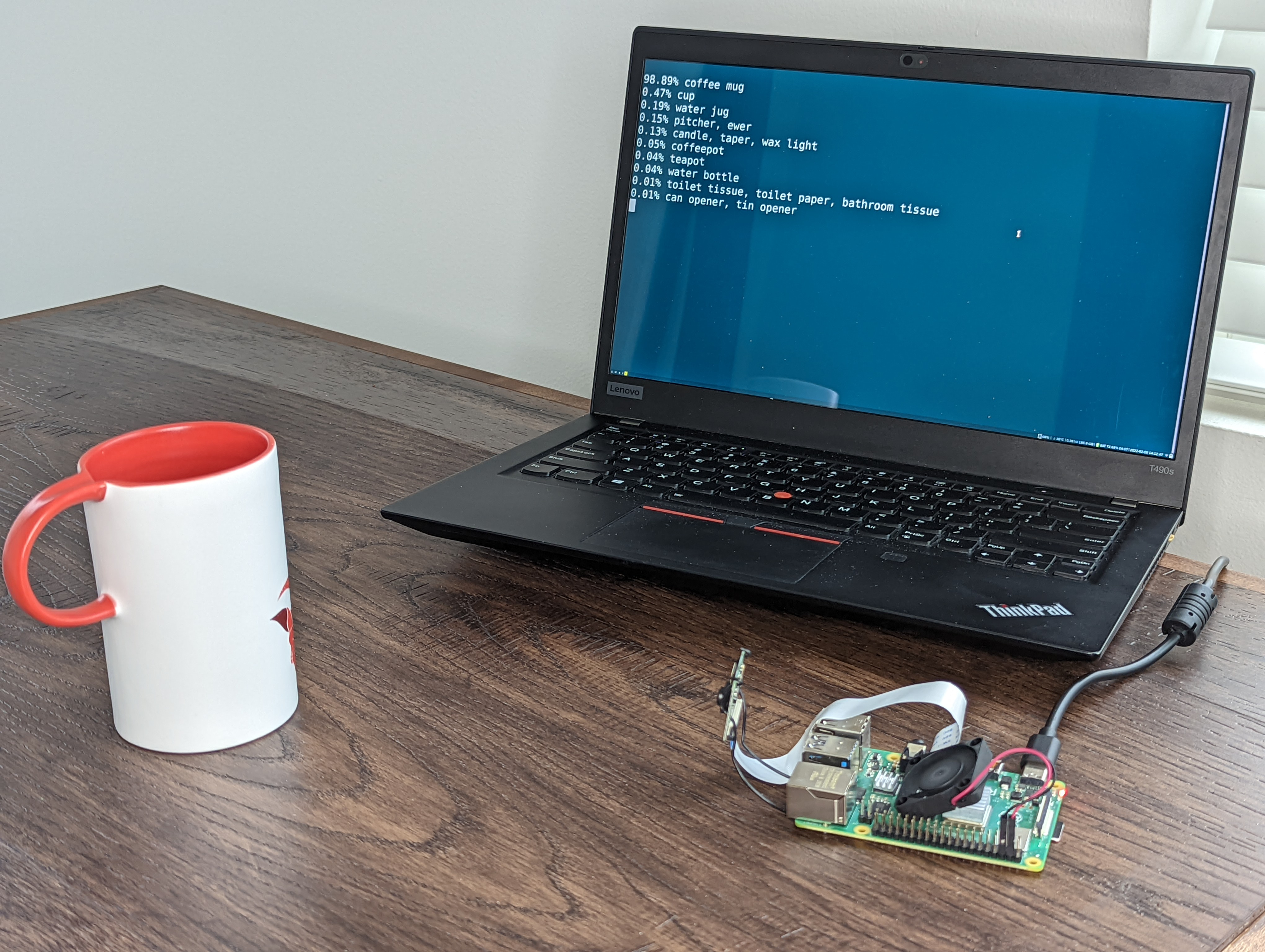# 故障排除：性能

torch.set_num_threads(2)


# 后续步骤

• 量化，了解有关如何量化和融合模型的详细信息。

• 迁移学习教程，介绍如何使用迁移学习将预先存在的模型微调到数据集。